# “Easy” Portfolio Allocation

This is an elementary mathematical finance article. This means if you know some math (linear algebra, differential calculus) you can find a quick solution to a simple finance question. The topic was inspired by a recent article in The American Mathematical Monthly (Volume 117, Number 1 January 2010, pp. 3-26): “Find Good Bets in the Lottery, and Why You Shouldn’t Take Them” by Aaron Abrams and Skip Garibaldi which said optimal asset allocation is now an undergraduate exercise. That may well be, but there are a lot of people with very deep mathematical backgrounds that have yet to have seen this. We will fill in the details here. The style is terse, but the content should be about what you would expect from one day of lecture in a mathematical finance course.

Portfolio allocation is not the “magic predict the future” part of finance, it is the scheme for correctly applying magic predictions of the future. The idea is that if you had an prediction of future returns of a number of assets, the naive thing to do would be to invest everything into the asset with highest predicted return. Portfolio theory, while still taking the predictions at face value, picks an investment pattern that will (in risk-adjusted dollars) outperform the naive strategy even if the predictions are correct and is a bit safer when the predictions are wrong.

Suppose you haddifferent assets you could invest in. For the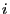-th asset there is an expected excess relative return ofand an estimated variance of(for a definition of relative return see Relative returns: a banker versus trader paradox and for a definition of variance see A Quick Appreciation of the Sharpe Ratio). Let the vectorbe such thatrepresents the number of dollars we invest in the-th asset. Ifis positive then our plan is “to go long” or buy some of the-th asset. Ifis negative our plan is “to short” or sell some of the-th asset to somebody else (It is called going short as we actually sell something we do not have. This is often allowed in finance; as long as we make the same pay-outs to the buyer that the buyer would receive if we really had the item to sell).

When we appeal to the idea of optimizing the portfolio Sharpe Ratio (again, see A Quick Appreciation of the Sharpe Ratio) then we say a good portfolio is one that doesn’t just maximize expected relative returns (which is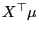) but maximizes the ratio of expected relative return to standard deviation:where (for now)is the matrix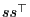. This ratio is called a “risk adjusted return” (versus the un-adjusted form). Also notice that the ratio is homogeneous in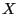(doublingdoes not change the ratio as it simultaneously doubles the numerator and the denominator) so an optimal solutiondescribes not how much to invest, but what pattern to invest in. This allows us to introduce an important practical constraint: we are only going to allow ourselves to risk a total ofdollars (both long and short). That is: we insist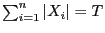. We will ignore this total investment constraint until the end when we can satisfy the constraint by simply re-scaling an partial solution.

To solve forwe introduce an old friend: Lagrange Multipliers (or equivalently the Karush-Kuhn-Tucker conditions of optimality). Since the fraction we are trying to optimize is homogeneous inwe can convert the denominator into a constraint and arbitrarily insist thatwithout changing the nature of the problem. We are now trying to maximizesubject to. The Lagrangian conditions of optimality state at the optimum we must have the gradient of the objective is proportional to the gradient of the constraint or:for some (to be determined) constant. Pushing the gradient operator through we get: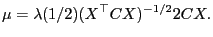A similar equation could be gotten by appealing to a Rayleigh Quotient argument.

We do not yet know(that is what we are trying to solve for), so we do not know what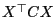is. However, this is just a scalar and since we are just trying to solve up to a multiple we can throw it out and introduce a new multiple and see that it is enough to solve:where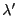is new (still unknown) scalar. This means we have:so our desired solution is some re-scaling of.

As we stated earlier we have a total investment constraint of. We can achieve this with the following adjusted solution:as our desired optimal portfolio allocation. In the end we can solve for the optimal portfolio by merely solving a linear system (we don’t need anything as expensive as a general purpose optimizer in this case).

These are very old results (going back as long as there has been Sharpe Ratios and portfolio theory). A good example reference is: “The Valuation of Risk Assets and the Selection of Risky Investments in Stock Portfolios and Capital Budgets,” John Lintner, The Review of Economics and Statistics (1965) vol. 47 (1) pp. 13-37. These results are the basis for advice like: “diversify.” Without modeling risk you would tend to put all of your money in the predicted highest paying asset. When modeling risk you tend to put some of your money in each high paying asset and as long as they do not all fail at the same time you have some safety. Another (very different) route to diversification is the Kelly Criterion (discussed in What is the gambler’s equivalent of Amdahl’s Law?).

A very important risk we have not yet modeled is that our assets may have a tendency to fail at the same time (meaning we may not have really diversified usefully). The notion of assets may fail at the same time brings us to the ideas of correlation and covariance. When we tookwe were implicitly assuming (or modeling), without justification, that each possible asset was independent of all the others (that there was no correlation between asset returns). This is, of course, not going to be anywhere near true in practice. Instead we should taketo be the Covariance Matrix that represent our estimate of the assent to asset correlations. In this case the solution methods above all work exactly as before. Companies such as MSCI Barra have made complete businesses out of producing and selling estimates of.

Another issue is when we do not allow ourselves to “short” (or take a negative allocation of) assets. In this case we have the additional constraints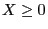which complicates our solution. For the special case where the asset variances are assumed to be independent (i.e.) it is enough to solve as above and merely replace any negative allocations with zero when inspecting and scaling the final step of the solution. When the covariances are non-trivial (has non-zero off-diagonal entries) this solution may not be optimal. In this case the Karush-Kuhn-Tucker conditions are more complicated and at the point of optimal solution we have the following conditions: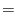00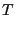00

whereis the allocation vector we wish to solve for,is an unknown scalar,is a new unknown vector andis the vector with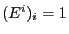and zeroes elsewhere. Using the Karush-Kuhn-Tucker conditions has allowed us to again almost linearize the problem, but we know have sign constraints onandand what is called a complementarity constraint:. This sort of problem essentially called a “Linear Complementarity Problem” and is about as hard as solving a linear program (the typical solution method is a variation of the simplex method called “Lemke’s algorithm”). (Technically theprevents the problem from being in the right form, butcan be inspected out of the problem.) The problem can still be solved, you just need a bit more software. If we can not short assets (or at least simulate shorting assets) we not only eliminate many possible portfolios from consideration (so we likely end up with a less profitable portfolio than we would like) we also make the mathematics and computation a bit harder.

The goal of this writeup has been to show how to systematically convert investment advice like “this stock is going to really take off” into an allocation of assets (which in turn implies a pattern of trades). We take as unexamined premises where to get such advice and whether to use the Sharpe ratio or some other notion of risk and/or utility. The point is that even though it may be complicated, from this point it is just calculation and calculation is easy to automate.

Categories: Mathematics Tutorials### jmount

Data Scientist and trainer at Win Vector LLC. One of the authors of Practical Data Science with R.